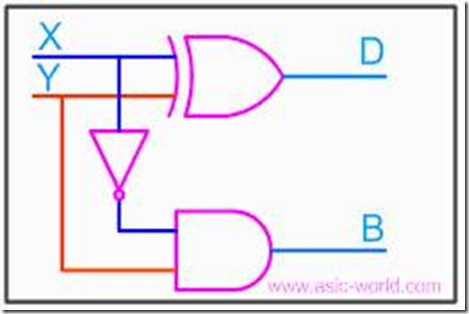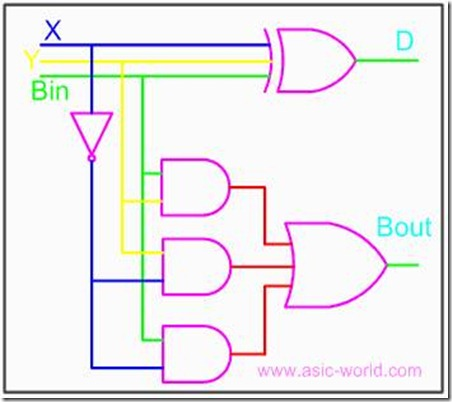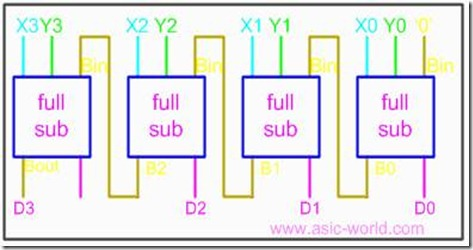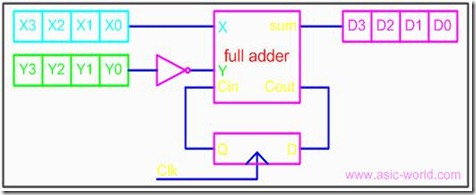### Digital Logic Circuits–Half and Full Subtractor

Subtractor

Subtractor circuits take two binary numbers as input and subtract one binary number input from the other binary number input. Similar to adders, it gives out two outputs, difference and borrow (carry-in the case of Adder). There are two types of subtractors.

• Half Subtractor
• Full Subtractor

Half Subtractor

The half-subtractor is a combinational circuit which is used to perform subtraction of two bits. It has two inputs, X (minuend) and Y (subtrahend) and two outputs D (difference) and B (borrow). The logic symbol and truth table are shown below.

Symbol

Truth Table

 X Y D B 0 0 0 0 0 1 1 1 1 0 1 0 1 1 0 0

From the above table we can draw the Kmap as shown below for "difference" and " borrow". The boolean expression for the difference and Borrow can be written.

From the equation we can draw the half-subtractor as shown in the figure below.Full Subtractor

A full subtractor is a combinational circuit that performs subtraction involving three bits, namely minuend, subtrahend, and borrow-in. The logic symbol and truth table are shown below.

Symbol

Truth Table

 X Y Bin D Bout 0 0 0 0 0 0 0 1 1 1 0 1 0 1 1 0 1 1 0 1 1 0 0 1 0 1 0 1 0 0 1 1 0 0 0 1 1 1 1 1

From above table we can draw the Kmap as shown below for "difference" and "borrow".

The boolean expression for difference and borrow can be written as

D = X'Y'Bin + X'YBin' + XY'Bin' + XYBin

= (X'Y' + XY)Bin + (X'Y + XY')Bin'

Bout = X'.Y + X'.Bin + Y.Bin

From the equation we can draw the full-subtractor as shown in figure below.

Full-subtractor circuit is more or less same as a full-adder with slight modification.Parallel Binary Subtractor

Parallel binary subtractor can be implemented by cascading several full-subtractors. Implementation and associated problems are those of a parallel binary adder, seen before in parallel binary adder section.

Below is the block level representation of a 4-bit parallel binary subtractor, which subtracts 4-bit Y3Y2Y1Y0 from 4-bit X3X2X1X0. It has 4-bit difference output D3D2D1D0 with borrow output Bout.A serial subtractor can be obtained by converting the serial adder using the 2's complement system. The subtrahend is stored in the Y register and must be 2's complemented before it is added to the minuend stored in the X register.

The circuit for a 4-bit serial subtractor using full-adder is shown in the figure below.